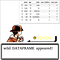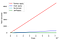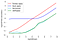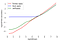# Swifter — automatically efficient pandas apply operationsWhat do you do?

# swifter

`\$ pip install -U pandas\$ pip install swifter`
`import pandas as pdimport swifter`
`myDF['outCol'] = DF['inCol'].swifter.apply(anyfunction)`

# Examples

`def bikes_proportion(x, max_x):    return x * 1.0 / max_xdata['bike_prop'] = data['bikes_available'].swifter.apply(                    bikes_proportion,                    max_x=np.max(data['bikes_available']))`
`def convert_to_human(datetime):    return datetime.weekday_name + ', the ' + str(datetime.day) + 'th day of ' + datetime.strftime("%B") + ', ' + str(datetime.year)data['humanreadable_date'] = data['date'].swifter.apply(                             convert_to_human)`
`# Parallel processing b/c if-else statement makes it non-vectorizeddef gt_5_bikes(x):    if x > 5:        return True    else:        return False# computes in 13.8sdata['gt_5_bikes'] = data['bikes_available'].swifter.apply(gt_5_bikes)# Vectorized versiondef gt_5_bikes_vectorized(x):    return np.where(x > 5, True, False)# computes in 231msdata['gt_5_bikes_vec'] = data['bikes_available'].swifter.apply(                         gt_5_bikes_vectorized)`

# BenchmarksSwifter vectorizes when possible for ≥100x speed increase
`df['date'].apply(pd.to_datetime) # very slowpd.to_datetime(df['date']) # vectorized - very fastdf['date'].swifter.apply(pd.to_datetime) # also vectorized - very fast`X is 1, 10, 100, 1000, …Swifter converges to pandas apply on small datasets and dask parallel processing on large ones

Written by

## Jason Carpenter

#### Machine Learning Engineer @ Manifold.ai

Welcome to a place where words matter. On Medium, smart voices and original ideas take center stage - with no ads in sight. Watch
Follow all the topics you care about, and we’ll deliver the best stories for you to your homepage and inbox. Explore
Get unlimited access to the best stories on Medium — and support writers while you’re at it. Just \$5/month. Upgrade